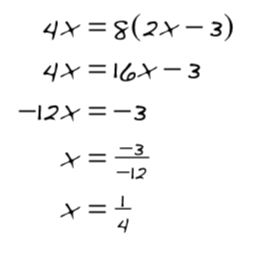Home > CCA > Chapter 4 > Lesson 4.3.1 > Problem4-102

4-102.

Mr. Greer solved an equation below. However, when he checked his solution, it did not make the original equation true. Find his error and then find the correct solution. Homework Help ✎Check Mr. Greer's distribution. Did he do it correctly?

Is $8$ times $−3$ equal to $−3$?

Solve the equation by distributing correctly.